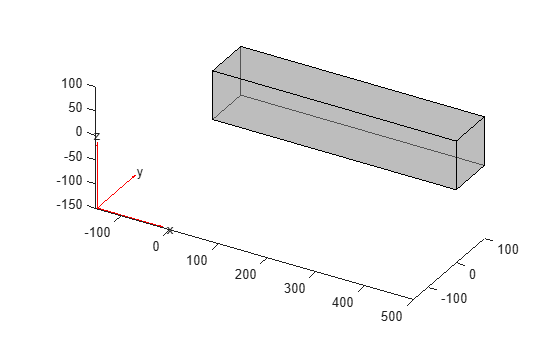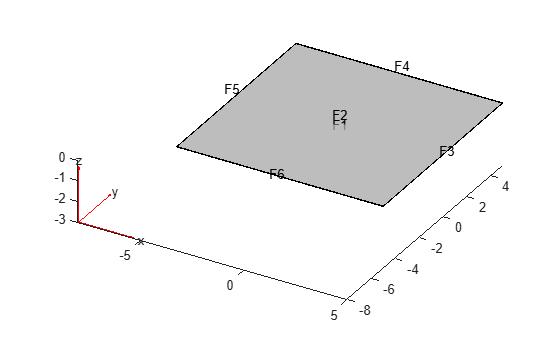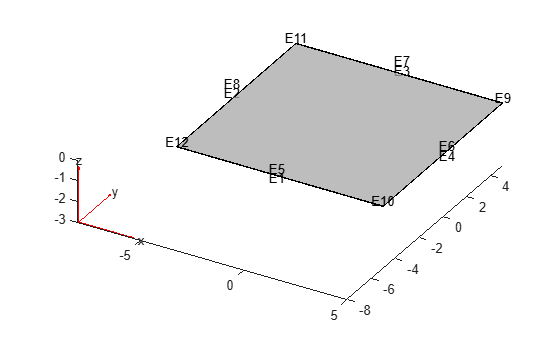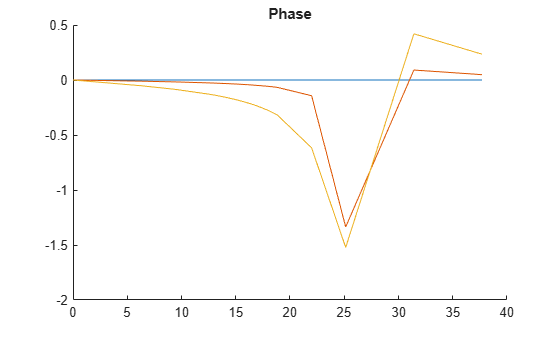structuralDamping

Specify damping parameters for transient or frequency response structural model

Description

example

structuralDamping(structuralmodel,'Alpha',a,'Beta',b) specifies proportional (Rayleigh) damping parameters a and b for a structuralmodel object.

For a frequency response model with damping, the results are complex. Use the abs and angle functions to obtain real-valued magnitude and phase, respectively.

example

structuralDamping(structuralmodel,'Zeta',z) specifies the modal damping ratio. Use this parameter when you solve a transient or frequency response model using the results of modal analysis.

damping = structuralDamping(___) returns the damping parameters object, using any of the previous input syntaxes.

Examples

collapse all

Specify proportional (Rayleigh) damping parameters for a beam.

Create a transient structural model.

structuralModel = createpde('structural','transient-solid');

Import and plot the geometry.

gm = importGeometry(structuralModel,'SquareBeam.stl');
pdegplot(structuralModel,'FaceAlpha',0.5)Specify the Young's modulus, Poisson's ratio, and mass density.

structuralProperties(structuralModel,'YoungsModulus',210E9,...
'PoissonsRatio',0.3,...
'MassDensity',7800);

Specify the Rayleigh damping parameters.

structuralDamping(structuralModel,'Alpha',10,'Beta',2)
ans =
StructuralDampingAssignment with properties:

RegionType: 'Cell'
RegionID: 1
DampingModel: "proportional"
Alpha: 10
Beta: 2
Zeta: []

Solve a frequency response problem with damping. The resulting displacement values are complex. To obtain the magnitude and phase of displacement, use the abs and angle functions, respectively. To speed up computations, solve the model using the results of modal analysis.

Modal Analysis

Create a modal analysis model for a 3-D problem.

modelM = createpde('structural','modal-solid');

Create the geometry and include it in the model.

gm = multicuboid(10,10,0.025);
modelM.Geometry = gm;

Generate a mesh.

msh = generateMesh(modelM);

Specify the Young's modulus, Poisson's ratio, and mass density of the material.

structuralProperties(modelM,'YoungsModulus',2E11, ...
'PoissonsRatio',0.3, ...
'MassDensity',8000);

Identify faces for applying boundary constraints and loads by plotting the geometry with the face and edge labels.

pdegplot(gm,'FaceLabels','on','FaceAlpha',0.5)figure
pdegplot(gm,'EdgeLabels','on','FaceAlpha',0.5)Specify constraints on the sides of the plate (faces 3, 4, 5, and 6) to prevent rigid body motions.

structuralBC(modelM,'Face',[3,4,5,6],'Constraint','fixed');

Solve the problem for the frequency range from -Inf to 12*pi.

Rm = solve(modelM,'FrequencyRange',[-Inf,12*pi]);

Frequency Response Analysis

Create a frequency response analysis model for a 3-D problem.

modelFR = createpde('structural','frequency-solid');

Use the same geometry and mesh as you used for the modal analysis.

modelFR.Geometry = gm;
modelFR.Mesh = msh;

Specify the same values for the Young's modulus, Poisson's ratio, and mass density of the material.

structuralProperties(modelFR,'YoungsModulus',2E11, ...
'PoissonsRatio',0.3, ...
'MassDensity',8000);

Specify the same constraints on the sides of the plate to prevent rigid body modes.

structuralBC(modelFR,'Face',[3,4,5,6],'Constraint','fixed');

Specify the pressure loading on top of the plate (face 2) to model an ideal impulse excitation. In the frequency domain, this pressure pulse is uniformly distributed across all frequencies.

First, solve the model without damping.

flist = [0,1,1.5,linspace(2,3,100),3.5,4,5,6]*2*pi;
RfrModalU = solve(modelFR,flist,'ModalResults',Rm);

Now, solve the model with damping equal to 2% of critical damping for all modes.

structuralDamping(modelFR,'Zeta',0.02);
RfrModalAll = solve(modelFR,flist,'ModalResults',Rm);

Solve the same model with frequency-dependent damping. In this example, use the solution frequencies from flist and damping values between 1% and 10% of critical damping.

omega = flist;
zeta = linspace(0.01,0.1,length(omega));
zetaW = @(omegaMode) interp1(omega,zeta,omegaMode);
structuralDamping(modelFR,'Zeta',zetaW);

RfrModalFD = solve(modelFR,flist,'ModalResults',Rm);

Interpolate the displacement at the center of the top surface of the plate for all three cases.

iDispU = interpolateDisplacement(RfrModalU,[0;0;0.025]);
iDispAll = interpolateDisplacement(RfrModalAll,[0;0;0.025]);
iDispFD = interpolateDisplacement(RfrModalFD,[0;0;0.025]);

Plot the magnitude of the displacement. Zoom in on the frequencies around the first mode.

figure
hold on
plot(RfrModalU.SolutionFrequencies,abs(iDispU.Magnitude));
plot(RfrModalAll.SolutionFrequencies,abs(iDispAll.Magnitude));
plot(RfrModalFD.SolutionFrequencies,abs(iDispFD.Magnitude));
title('Magnitude')
xlim([10 25])
ylim([0 0.5])Plot the phase of the displacement.

figure
hold on
plot(RfrModalU.SolutionFrequencies,angle(iDispU.Magnitude));
plot(RfrModalAll.SolutionFrequencies,angle(iDispAll.Magnitude));
plot(RfrModalFD.SolutionFrequencies,angle(iDispFD.Magnitude));
title('Phase')Input Arguments

collapse all

Transient or frequency response structural model, specified as a StructuralModel object. The model contains the geometry, mesh, structural properties of the material, body loads, boundary loads, boundary conditions, and initial conditions.

Example: structuralmodel = createpde('structural','transient-solid')

Mass proportional damping, specified as a nonnegative number.

Data Types: double

Stiffness proportional damping, specified as a nonnegative number.

Data Types: double

Modal damping ratio, specified as a nonnegative number or a function handle. Use a function handle when each mode has its own damping ratio. The function must accept a vector of natural frequencies as an input argument and return a vector of corresponding damping ratios. It must cover the full frequency range for all modes used for modal solution. For details, see Modal Damping Depending on Frequency.

Data Types: double | function_handle

Output Arguments

collapse all

Handle to damping parameters, returned as a StructuralDampingAssignment object. See StructuralDampingAssignment Properties.

collapse all

Modal Damping Depending on Frequency

To use the individual value of modal damping for each mode, specify z as a function of frequency.

function z = dampingFcn(omega)

Typically, the damping ratio function is a linear interpolation of frequency versus the modal damping parameter:

structuralDamping(modelD,'Zeta',@(omegaMode) ...
interp1(omega,zeta,omegaMode))

Here, omega is a vector of frequencies, and zeta is a vector of corresponding damping ratio values.

Introduced in R2018a

Partial Differential Equation Toolbox DocumentationGet trial now